## Koch Snowflake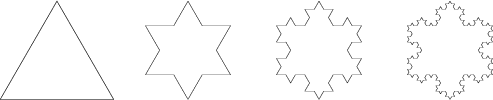A Fractal, also known as the Koch Island, which was first described by Helge von Koch in 1904. It is built by starting with an Equilateral Triangle, removing the inner third of each side, building another Equilateral Triangle at the location where the side was removed, and then repeating the process indefinitely. The Koch snowflake can be simply encoded as a Lindenmayer System with initial string "F-F-F", String Rewriting rule "F" -> "F+F-F+F", and angle 60°. The zeroth through third iterations of the construction are shown above. The fractal can also be constructed using a base curve and motif, illustrated below.Let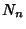be the number of sides,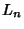be the length of a single side,be the length of the Perimeter, and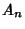the snowflake's Area after theth iteration. Further, denote the Area of the initialTriangle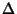, and the length of an initialside 1. Then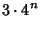(1)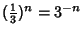(2)(3)(4)

The Capacity Dimension is then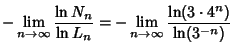(5)

Now compute the Area explicitly,(6)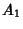(7)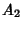(8)(9)

so as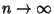,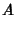(10)Some beautiful Tilings, a few examples of which are illustrated above, can be made with iterations toward Koch snowflakes.In addition, two sizes of Koch snowflakes in Area ratio 1:3 Tile the Plane, as shown above (Mandelbrot).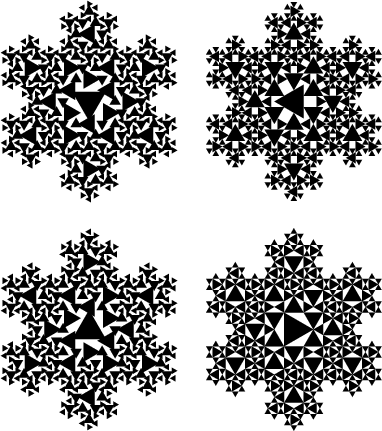Another beautiful modification of the Koch snowflake involves inscribing the constituent triangles with filled-in triangles, possibly rotated at some angle. Some sample results are illustrated above for 3 and 4 iterations.

See also Cesàro Fractal, Exterior Snowflake, Gosper Island, Koch Antisnowflake, Peano-Gosper Curve, Pentaflake, Sierpinski Sieve

References

Cundy, H. and Rollett, A. Mathematical Models, 3rd ed. Stradbroke, England: Tarquin Pub., pp. 65-66, 1989.

Dickau, R. M. Two-Dimensional L-Systems.'' http://forum.swarthmore.edu/advanced/robertd/lsys2d.html.

Dixon, R. Mathographics. New York: Dover, pp. 175-177 and 179, 1991.

Lauwerier, H. Fractals: Endlessly Repeated Geometric Figures. Princeton, NJ: Princeton University Press, pp. 28-29 and 32-36, 1991.

Pappas, T. The Snowflake Curve.'' The Joy of Mathematics. San Carlos, CA: Wide World Publ./Tetra, pp. 78 and 160-161, 1989.

Peitgen, H.-O.; Jürgens, H.; and Saupe, D. Chaos and Fractals: New Frontiers of Science. New York: Springer-Verlag, 1992.

Peitgen, H.-O. and Saupe, D. (Eds.). The von Koch Snowflake Curve Revisited.'' §C.2 in The Science of Fractal Images. New York: Springer-Verlag, pp. 275-279, 1988.

Wagon, S. Mathematica in Action. New York: W. H. Freeman, pp. 185-195, 1991.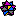Weisstein, E. W. Fractals.'' Mathematica notebook Fractal.m.